## Numerical integration of Ordinary Differential Equations¶

This notebook serves as a quick refresher on ordinary differential equations. If you are familiar with the topic: feel free to skim this notebook.

We will first consider the decay of tritium as an example:

$$\mathrm{^3H \overset{\lambda}\rightarrow\ ^3He + e^- + \bar{\nu_e}}$$

We will not concern ourselves with the products, instead we will only take interest in the number density of $\mathrm{^3H}$ as function of time, let's call it $y(t)$. The rate of change of $y(t)$ is proportional to itself and the decay constant ($\lambda$):

$$\frac{dy(t)}{dt} = -\lambda y(t)$$

you probably know the solution to this class of differential equations (either from experience or by guessing an appropriate ansatz). SymPy can of course also solve this equation:

In :
import sympy as sym
sym.init_printing()
t, l = sym.symbols('t lambda')
y = sym.Function('y')(t)
dydt = y.diff(t)
expr = sym.Eq(dydt, -l*y)
expr

Out:
$$\frac{d}{d t} y{\left (t \right )} = - \lambda y{\left (t \right )}$$
In :
sym.dsolve(expr)

Out:
$$y{\left (t \right )} = C_{1} e^{- \lambda t}$$

Now, pretend for a while that this function lacked an analytic solution. We could then integrate this equation numerically from an initial state for a predetermined amount of time by discretizing the time into a seriers of small steps.

### Explicit methods¶

For each step taken we would update $y$ by multiplying the derivative with the step size (assuming that the derivate is approximately constant on the scale of the step-size), formally this method is known as "forward Euler":

$$y_{n+1} = y_n + y'(t_n)\cdot \Delta h$$

this is known as an explicit method, i.e. the derivative at the current time step is used to calculate the next step forward.

For demonstration purposes only, we implement this in Python:

In :
import numpy as np
def euler_fw(rhs, y0, tout, params):
y0 = np.atleast_1d(np.asarray(y0, dtype=np.float64))
dydt = np.empty_like(y0)
yout = np.zeros((len(tout), len(y0)))
yout = y0
t_old = tout
for i, t in enumerate(tout[1:], 1):
dydt[:] = rhs(yout[i-1], t, *params)
h = t - t_old
yout[i] = yout[i-1] + dydt*h
t_old = t
return yout


applying this function on our model problem:

In :
def rhs(y, t, decay_constant):
return -decay_constant*y  # the rate does not depend on time ("t")

In :
tout = np.linspace(0, 2e9, 100)
y0 = 3
params = (1.78e-9,)  # 1 parameter, decay constant of tritium
yout = euler_fw(rhs, y0, tout, params)


and plotting the solution & the numerical error using matplotlib:

In :
import matplotlib.pyplot as plt
%matplotlib inline

def my_plot(tout, yout, params, xlbl='time / a.u.', ylabel=None, analytic=None):
fig, axes = plt.subplots(1, 2 if analytic else 1, figsize=(14, 4))
axes = np.atleast_1d(axes)
for i in range(yout.shape):
axes.plot(tout, yout[:, i], label='y%d' % i)
if ylabel:
axes.set_ylabel(ylabel)
for ax in axes:
ax.set_xlabel(xlbl)
if analytic:
axes.plot(tout, analytic(tout, yout, params), '--')
axes.plot(tout, yout[:, 0] - yout*np.exp(-params*(tout-tout)))
if ylabel:
axes.set_ylabel('Error in ' + ylabel)

In :
def analytic(tout, yout, params):
return yout[0, 0]*np.exp(-params*tout)
my_plot(tout, yout, params, analytic=analytic, ylabel='number density / a.u.')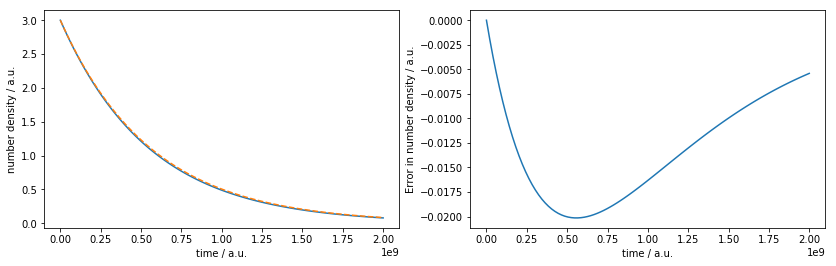We see that 100 points gave us almost plotting accuracy.

Unfortunately, Euler forward is not practical for most real world problems. Usually we want a higher order formula (the error in Euler forward scales only as $n^{-1}$), and we want to use an adaptive step size (larger steps when the function is smooth). So we use the well tested LSODA algorithm (provided in scipy as odeint):

In :
from scipy.integrate import odeint
yout, info = odeint(rhs, y0, tout, params, full_output=True)
my_plot(tout, yout, params, analytic=analytic)
print("Number of function evaluations: %d" % info['nfe'][-1])

Number of function evaluations: 95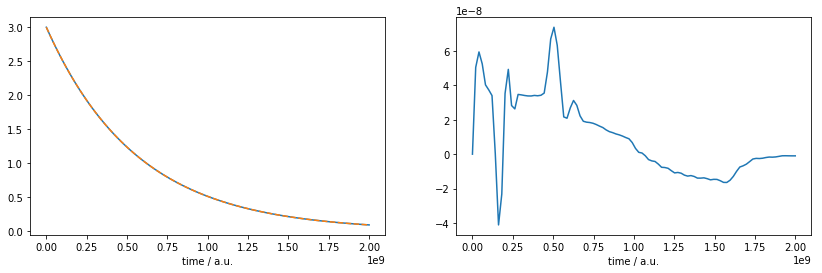We can see that odeint was able to achieve a much higher precision using fewer number of function evaluations.

### Implicit methods¶

For a large class of problems we need to base the step not on the derivative at the current time point, but rather at the next one (giving rise to an implicit expression). The simplest implicit stepper is "backward euler":

$$y_{n+1} = y_n + y'(t_{n+1})\cdot \Delta h$$

Problems requiring this type of steppers are known as "stiff". We will not go into the details of this (LSODA actually uses something more refined and switches between explicit and implicit steppers).

In the upcoming notebooks we will use odeint to solve systems of ODEs (and not only linear equations as in this notebook). The emphasis is not on the numerical methods, but rather on how we, from symbolic expressions, can generate fast functions for the solver.

### Systems of differential equations¶

In order to show how we would formulate a system of differential equations we will here briefly look at the van der Pol osciallator. It is a second order differential equation:

$${d^2y_0 \over dx^2}-\mu(1-y_0^2){dy_0 \over dx}+y_0= 0$$

One way to reduce the order of our second order differential equation is to formulate it as a system of first order ODEs, using:

$$y_1 = \dot y_0$$

which gives us:

$$\begin{cases} \dot y_0 = y_1 \\ \dot y_1 = \mu(1-y_0^2) y_1-y_0 \end{cases}$$

Let's call the function for this system of ordinary differential equations vdp:

In :
def vdp(y, t, mu):
return [
y,
mu*(1-y**2)*y - y
]


using "Euler forward":

In :
tout = np.linspace(0, 200, 1024)
y_init, params = [1, 0], (17,)
y_euler = euler_fw(vdp, y_init, tout, params)  # never mind the warnings emitted here...

/home/travis/miniconda3/envs/codegen17/lib/python3.6/site-packages/ipykernel_launcher.py:4: RuntimeWarning: overflow encountered in double_scalars
after removing the cwd from sys.path.
/home/travis/miniconda3/envs/codegen17/lib/python3.6/site-packages/ipykernel_launcher.py:11: RuntimeWarning: invalid value encountered in add
# This is added back by InteractiveShellApp.init_path()

In :
my_plot(tout, y_euler, params)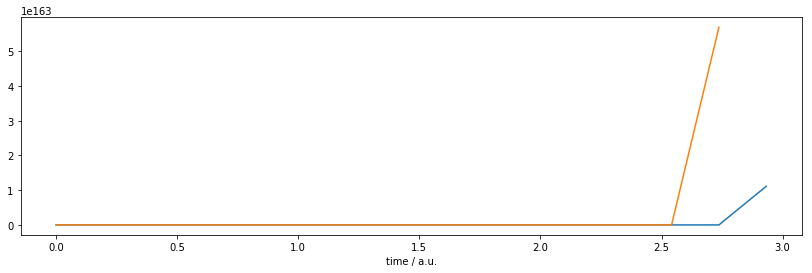That does not look like an oscillator. (we see that Euler forward has deviated to values with enormous magnitude), here the advanced treatment by the odeint solver is far superior:

In :
y_odeint, info = odeint(vdp, y_init, tout, params, full_output=True)
print("Number of function evaluations: %d, number of Jacobian evaluations: %d" % (info['nfe'][-1], info['nje'][-1]))
my_plot(tout, y_odeint, params)

Number of function evaluations: 9244, number of Jacobian evaluations: 211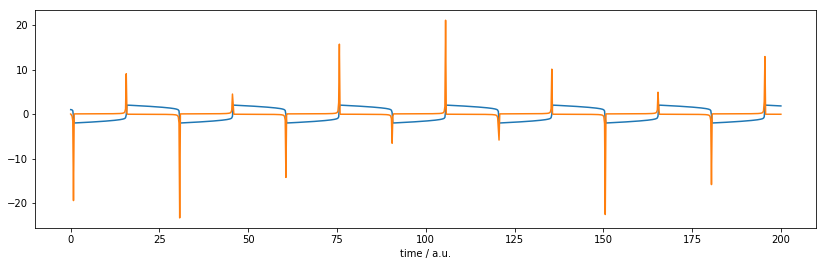We see that LSODA has evaluated the Jacobian. But we never gave it an explicit representation of it―so how could it?

It estimated the Jacobian matrix by using finite differences. Let's see if we can do better if we provide a function to calculate the (analytic) Jacobian.

## Exercise: manually write a function evaluating a Jacobian¶

First we need to know what signature odeint expects, we look at the documentation by using the help command: (or using ? in IPython)

In :
help(odeint)  # just skip to "Dfun"

Help on function odeint in module scipy.integrate.odepack:

odeint(func, y0, t, args=(), Dfun=None, col_deriv=0, full_output=0, ml=None, mu=None, rtol=None, atol=None, tcrit=None, h0=0.0, hmax=0.0, hmin=0.0, ixpr=0, mxstep=0, mxhnil=0, mxordn=12, mxords=5, printmessg=0)
Integrate a system of ordinary differential equations.

Solve a system of ordinary differential equations using lsoda from the
FORTRAN library odepack.

Solves the initial value problem for stiff or non-stiff systems
of first order ode-s::

dy/dt = func(y, t0, ...)

where y can be a vector.

*Note*: The first two arguments of func(y, t0, ...) are in the
opposite order of the arguments in the system definition function used
by the scipy.integrate.ode class.

Parameters
----------
func : callable(y, t0, ...)
Computes the derivative of y at t0.
y0 : array
Initial condition on y (can be a vector).
t : array
A sequence of time points for which to solve for y.  The initial
value point should be the first element of this sequence.
args : tuple, optional
Extra arguments to pass to function.
Dfun : callable(y, t0, ...)
Gradient (Jacobian) of func.
col_deriv : bool, optional
True if Dfun defines derivatives down columns (faster),
otherwise Dfun should define derivatives across rows.
full_output : bool, optional
True if to return a dictionary of optional outputs as the second output
printmessg : bool, optional
Whether to print the convergence message

Returns
-------
y : array, shape (len(t), len(y0))
Array containing the value of y for each desired time in t,
with the initial value y0 in the first row.
infodict : dict, only returned if full_output == True
Dictionary containing additional output information

=======  ============================================================
key      meaning
=======  ============================================================
'hu'     vector of step sizes successfully used for each time step.
'tcur'   vector with the value of t reached for each time step.
(will always be at least as large as the input times).
'tolsf'  vector of tolerance scale factors, greater than 1.0,
computed when a request for too much accuracy was detected.
'tsw'    value of t at the time of the last method switch
(given for each time step)
'nst'    cumulative number of time steps
'nfe'    cumulative number of function evaluations for each time step
'nje'    cumulative number of jacobian evaluations for each time step
'nqu'    a vector of method orders for each successful step.
'imxer'  index of the component of largest magnitude in the
weighted local error vector (e / ewt) on an error return, -1
otherwise.
'lenrw'  the length of the double work array required.
'leniw'  the length of integer work array required.
'mused'  a vector of method indicators for each successful time step:
1: adams (nonstiff), 2: bdf (stiff)
=======  ============================================================

Other Parameters
----------------
ml, mu : int, optional
If either of these are not None or non-negative, then the
Jacobian is assumed to be banded.  These give the number of
lower and upper non-zero diagonals in this banded matrix.
For the banded case, Dfun should return a matrix whose
rows contain the non-zero bands (starting with the lowest diagonal).
Thus, the return matrix jac from Dfun should have shape
(ml + mu + 1, len(y0)) when ml >=0 or mu >=0.
The data in jac must be stored such that jac[i - j + mu, j]
holds the derivative of the ith equation with respect to the jth
state variable.  If col_deriv is True, the transpose of this
jac must be returned.
rtol, atol : float, optional
The input parameters rtol and atol determine the error
control performed by the solver.  The solver will control the
vector, e, of estimated local errors in y, according to an
inequality of the form max-norm of (e / ewt) <= 1,
where ewt is a vector of positive error weights computed as
ewt = rtol * abs(y) + atol.
rtol and atol can be either vectors the same length as y or scalars.
Defaults to 1.49012e-8.
tcrit : ndarray, optional
Vector of critical points (e.g. singularities) where integration
care should be taken.
h0 : float, (0: solver-determined), optional
The step size to be attempted on the first step.
hmax : float, (0: solver-determined), optional
The maximum absolute step size allowed.
hmin : float, (0: solver-determined), optional
The minimum absolute step size allowed.
ixpr : bool, optional
Whether to generate extra printing at method switches.
mxstep : int, (0: solver-determined), optional
Maximum number of (internally defined) steps allowed for each
integration point in t.
mxhnil : int, (0: solver-determined), optional
Maximum number of messages printed.
mxordn : int, (0: solver-determined), optional
Maximum order to be allowed for the non-stiff (Adams) method.
mxords : int, (0: solver-determined), optional
Maximum order to be allowed for the stiff (BDF) method.

--------
ode : a more object-oriented integrator based on VODE.
quad : for finding the area under a curve.

Examples
--------
The second order differential equation for the angle theta of a
pendulum acted on by gravity with friction can be written::

theta''(t) + b*theta'(t) + c*sin(theta(t)) = 0

where b and c are positive constants, and a prime (') denotes a
derivative.  To solve this equation with odeint, we must first convert
it to a system of first order equations.  By defining the angular
velocity omega(t) = theta'(t), we obtain the system::

theta'(t) = omega(t)
omega'(t) = -b*omega(t) - c*sin(theta(t))

Let y be the vector [theta, omega].  We implement this system
in python as:

>>> def pend(y, t, b, c):
...     theta, omega = y
...     dydt = [omega, -b*omega - c*np.sin(theta)]
...     return dydt
...

We assume the constants are b = 0.25 and c = 5.0:

>>> b = 0.25
>>> c = 5.0

For initial conditions, we assume the pendulum is nearly vertical
with theta(0) = pi - 0.1, and it initially at rest, so
omega(0) = 0.  Then the vector of initial conditions is

>>> y0 = [np.pi - 0.1, 0.0]

We generate a solution 101 evenly spaced samples in the interval
0 <= t <= 10.  So our array of times is:

>>> t = np.linspace(0, 10, 101)

Call odeint to generate the solution.  To pass the parameters
b and c to pend, we give them to odeint using the args
argument.

>>> from scipy.integrate import odeint
>>> sol = odeint(pend, y0, t, args=(b, c))

The solution is an array with shape (101, 2).  The first column
is theta(t), and the second is omega(t).  The following code
plots both components.

>>> import matplotlib.pyplot as plt
>>> plt.plot(t, sol[:, 0], 'b', label='theta(t)')
>>> plt.plot(t, sol[:, 1], 'g', label='omega(t)')
>>> plt.legend(loc='best')
>>> plt.xlabel('t')
>>> plt.grid()
>>> plt.show()



so the signature needs to be: (state-vector, time, parameters) -> matrix

In :
%load_ext scipy2017codegen.exercise


Use either the %exercise or %load magic to get the exercise / solution respecitvely (i.e. delete the whole contents of the cell except for the uncommented magic command). Replace ??? with the correct expression.

Remember that our system is defined as: $$\begin{cases} \dot y_0 = y_1 \\ \dot y_1 = \mu(1-y_0^2) y_1-y_0 \end{cases}$$

In :
# %exercise exercise_jac_func.py
def J_func(y, t, mu):
return np.array([
[0, 1],
[-1-2*mu*y*y, mu*(1-y**2)]  # EXERCISE: -1-2*mu*y*y
])

In :
J_func(y_init, tout, params)

Out:
array([[ 0,  1],
[-1,  0]])
In :
y_odeint, info = odeint(vdp, y_init, tout, params, full_output=True, Dfun=J_func)

In :
my_plot(tout, y_odeint, params)
print("Number of function evaluations: %d, number of Jacobian evaluations: %d" % (info['nfe'][-1], info['nje'][-1]))

Number of function evaluations: 8557, number of Jacobian evaluations: 194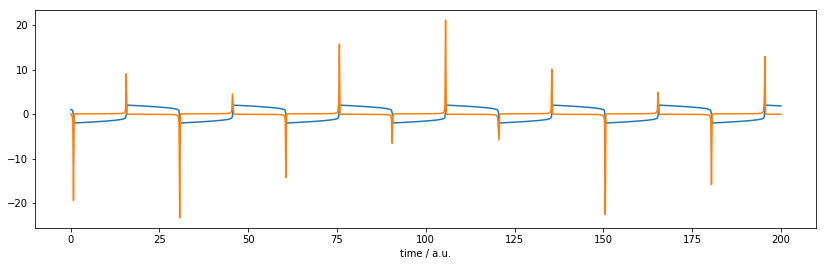So this time the integration needed to evaluate both the ODE system function and its Jacobian fewer times than when using finite difference approximations. The reason for this is that the more accurate the Jacobian is, the better is the convergence in the iterative (Newton's) method solving the implicit system of equations.

For larger systems of ODEs the importance of providing a (correct) analytic Jacobian can be much bigger.

### SymPy to the rescue¶

Instead of writing the jacobian function by hand we could have used SymPy's lambdify which we will introduce next. Here is a sneak peak on how it could be achieved:

In :
y = y0, y1 = sym.symbols('y0 y1')
mu = sym.symbols('mu')
J = sym.Matrix(vdp(y, None, mu)).jacobian(y)
J_func = sym.lambdify((y, t, mu), J)
J

Out:
$$\left[\begin{matrix}0 & 1\\- 2 \mu y_{0} y_{1} - 1 & \mu \left(- y_{0}^{2} + 1\right)\end{matrix}\right]$$